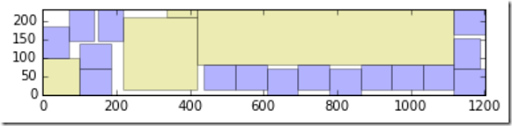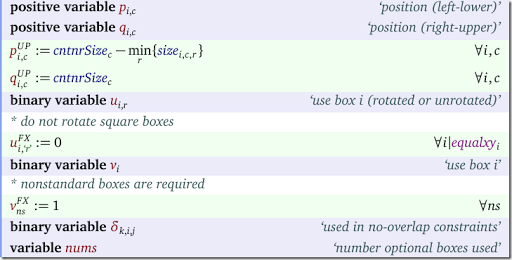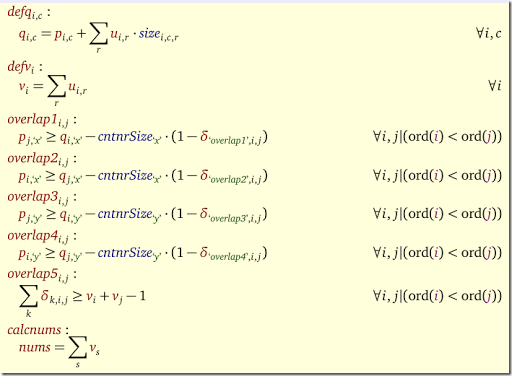## Sunday, June 19, 2016

### Filling up a container with boxes

In this post the following problem was proposed:

We have a rectangular container in which we pace some differently sized boxes (rectangles, this problem is 2D only). There is some space left, and we want to fill that with as many standard sized boxes as possible.Here the yellow boxes are the original pay load and the blue boxes are the ones we were able to add. Note that we are allowed to rotate the rectangles.

This can be formulated by a Mixed Integer Programming or a Constraint Programming model. The main ingredient is a set of ‘no-overlap’ constraints. Here is some background information about these constraints. In our case things are slightly more complex as we allow 90° rotation.

#### Variables

The decision variables we use are as follows:The variables $$q_{i,c}$$ are not really needed (we can recalculate them from $$p_{i,c}$$ and $$u_{i,r}$$ when needed) but they help to simplify a number of constraints.

#### EquationsThe model is not extremely complicated, although the rotation needs attention.

To get better performance we can add some symmetry-breaking constraints and use some branching priorities. Even then proving optimality is very difficult. However a good MIP solver will find very good solutions very quickly.